## Performance comparison of LSTM with and without cuDNN(v5) in Chainer

• By Motoki Sato
• Mar 15, 2017
• In General

We compare the performance of an LSTM network both with and without cuDNN in Chainer. The NVIDIA CUDA® Deep Neural Network library (cuDNN) is a GPU-accelerated library of primitives for deep neural networks. cuDNN provides highly tuned implementations for standard routines such as LSTM, CNN.

In this article, we compare the performance of LSTM with or without cuDNN. In Chainer, an LSTM implementation is configurable to run with or without cuDNN. An LSTM (NStepLSTM) implementation can be found here.

https://github.com/pfnet/chainer/blob/master/chainer/functions/connection/n_step_lstm.py

NVIDIA’s official blog is related to this post. Please check here.

### Summary:

Thorough our experiments, we found the following observations: We should use cuDNN

• if the model is large.
• if the sequence data is long.
• if the sequence data has variable lengths.

We conducted experiments from the following viewpoints:

• The effect of mini-batch size
• The effect of the number of LSTM layers and sequence length of data
• The effect of the number of LSTM layers and random sequence length of data
• The effect of the number of LSTM layers and the input unit size
• The effect of the number of LSTM layers and the hidden unit size
• The effect of the number of LSTM layers and dropout rate
• When will the differences be large? (setting with/without cuDNN)

### Experimental Result:

• The effect of mini-batch size parameters: batchsize = {127, 128, 200, 255, 256} In all results, batchsize 128 is faster than 127 and batchsize 256 is faster than 255. (Despite smaller batch size!) Using batchsize = 2^n will provide the best performance. Note that the number of iterations is the same number (39 iterations) in the case of batchsize = {255, 256}.

Comparing the setting with/without cuDNN, about 2 times ~ 2.8 times faster when using cuDNN in forward time, and 1.6 times faster in backward time.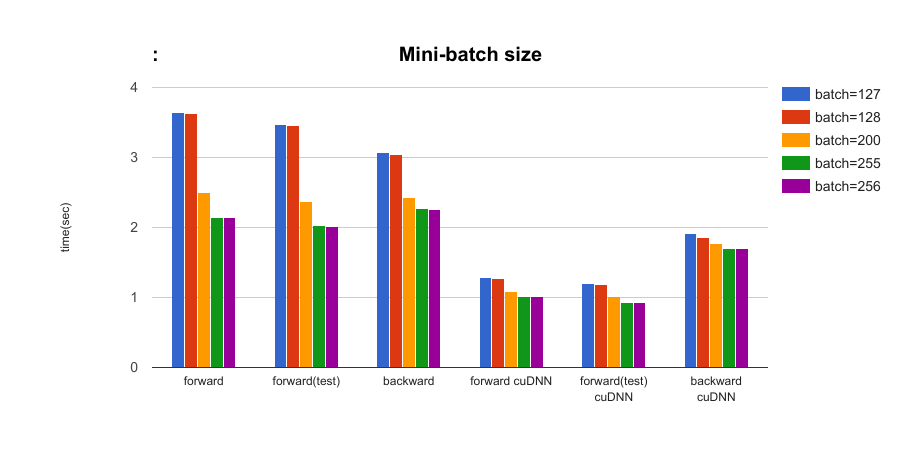• The effect of the layer size of LSTM and sequence length of data parameters: length={5, 25, 50}, layer={1, 2, 3} As the length of data and the number of layers increases, the performance benefit from the cuDNN implementation increases.

When we use a large LSTM setting (layer=3, length=50), cuDNN is about 7 times faster in forward time, and 4 times faster in backward time.

When we use a small LSTM setting (layer=1, length=5), about 1.5 times faster in forward time, and 1.04 times faster in backward time.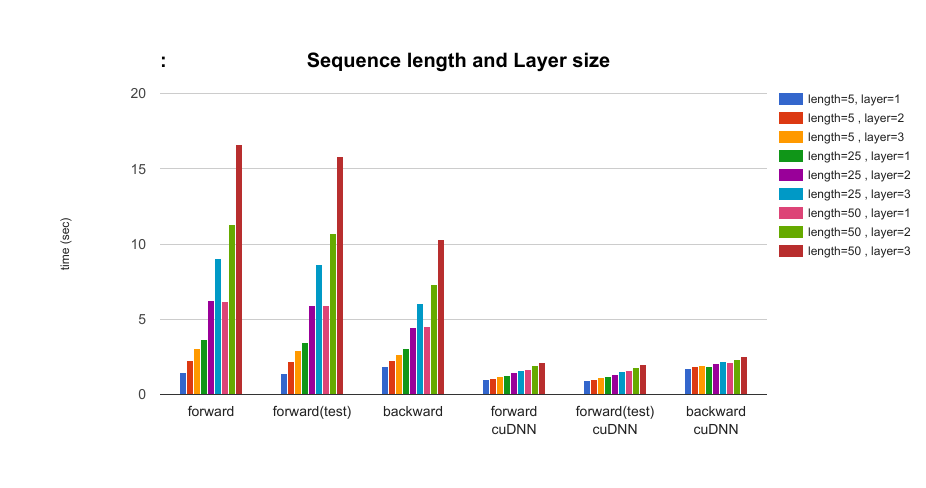• The effect of the layer size of LSTM and random sequence length of data parameters: layer = {1, 2, 3}, random={True, False} In this setting, we compare if the data sequence length is fixed or not. (Max length is 25.) When we use cuDNN, the performance impact of random sequence length is small.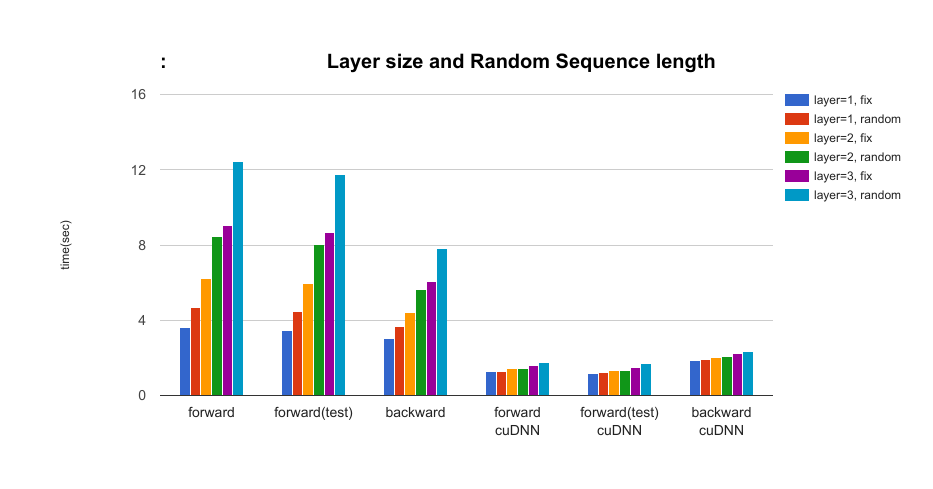• The effect of the layer size of LSTM and the input unit size parameters: layer = {1, 2, 3}, input={128, 256} In the setting with cuDNN, as number of layers increases, the difference between cuDNN and no-cuDNN will be large. (About 5 times faster in forward time, and about 2.7 times faster in backward time.)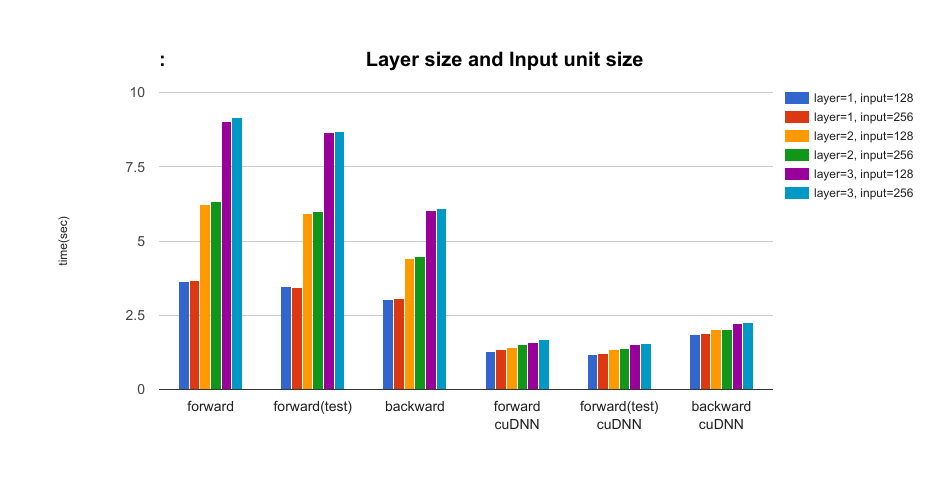• The effect of the layer size of LSTM and the hidden unit size parameters: layer = {1, 2, 3}, hidden={128, 256} In the setting with cuDNN, the number of layers increases, the difference between cuDNN and no-cuDNN will be large. (This is same as layer size and input size experiment.) However as hidden unit size increases, the difference between cuDNN and no-cuDNN will be small.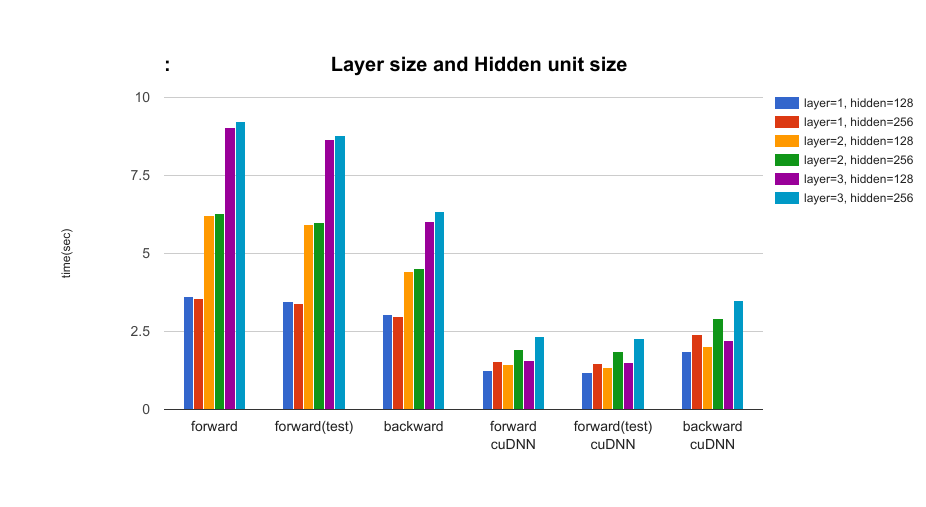• The effect of the layer size of LSTM and dropout rate parameters: layer={1, 2, 3}, dropout={0.5, 0.0} In the setting with cuDNN, when using dropout, the speed gets slower but the difference is very small (dropout rate=0.50).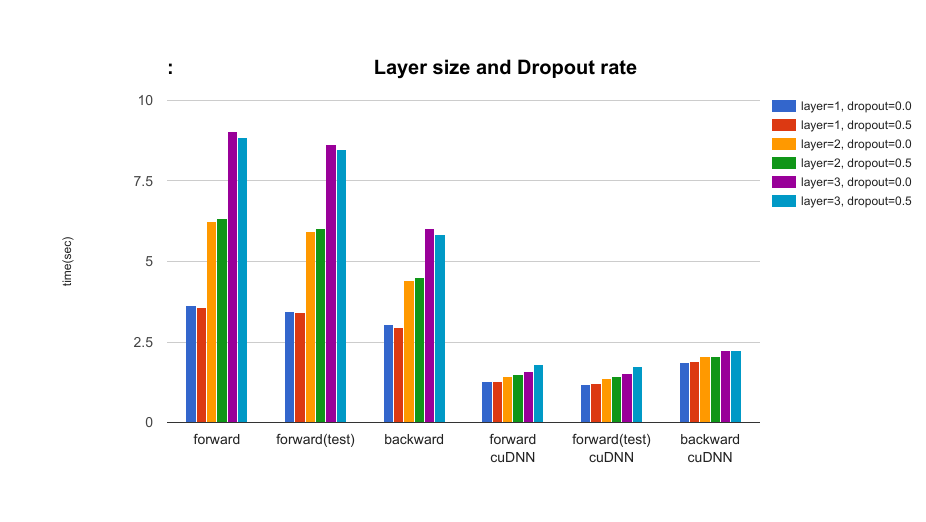• When will the differences be large? (Setting with/without cuDNN.)

As batch size is small (batchsize=128), sequence length is long (length=50) and the number of layers is large (layer=3), the difference is large. (The cuDNN is faster than no-cuDNN setting.)

If we use a large LSTM in the experiment, the performance benefit of using cuDNN will be large. 7.8 times faster in forward time. 4.0 times faster in backward time.

### Experimental Environment

• GPU: GeForce GTX 970
• Chainer (v1.21)
• cuDNN v5.1 (cuda v8)

### Experimental Setting

• Data: Random artificial sequence data (data size: 10,000)
• Training epoch: 10
• Comparing the average time per one epoch.
• Performance time of
• forward time (for train data)
• forward time (for test data)
• backward time
• Default experiment setting:
• batchsize : 128
• sequence length : 25
• random length : 0 (fix length)
• layer size: 1
• input unit size: 128
• hidden unit size : 128
• The code for our experiments:

https://github.com/aonotas/test-chainer-performance